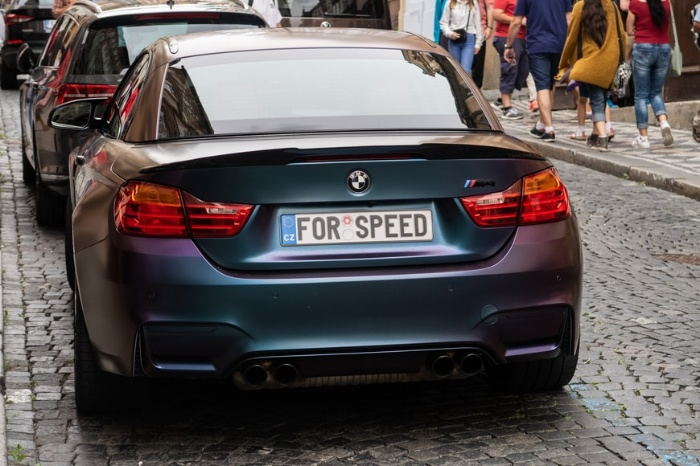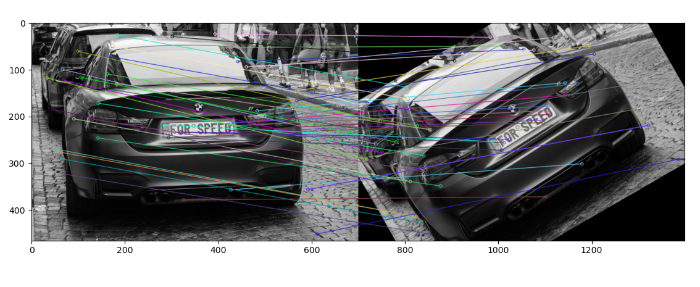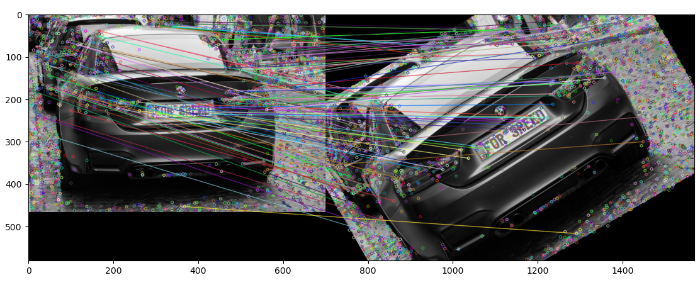# OpenCV Python – Implementing feature matching between two images using SIFT

We use Scale Invariant Feature Transform (SIFT) feature descriptor and Brute Force feature matcher to implement feature matching between two images. The SIFT is used to find the feature keypoints and descriptors in the images. A Brute Force matcher is used to match the descriptors in both images.

## Steps

To implement feature matching between two images using the SIFT feature detector and Brute Force matcher, you could follow the steps given below −

• Import required libraries OpenCV, Matplotlib and NumPy. Make sure you have already installed them.

• Read two input images using cv2.imread() method as grayscale images. Specify the full path of the image.

• Initiate SIFT object with default values using sift=cv2.SIFT_create().

• Detect and compute the keypoints 'kp1' & 'kp2' and descriptors 'des1' & 'des2' in the both input images using sift.detectAndCompute().

• Create a BFmatcher object bf = cv2.BFMatcher() and match the descriptors using this BFmatcher object as bf.match(des1, des2). It returns the matches. Sort the matches based on their distances.

• Draw the matches on the original input images using cv2.drawMatches().

• Optionally we can match the descriptors using the BFmatcher object method bf.knnMatch(des1,des2, k=2). Apply the ratio test on the matches to obtain best matches. Draw the matches using cv2.drawMatchesKnn().

• Visualize the keypoint matches.

Let's look at some examples to match keypoints of two images using the SIFT feature detector and Brute Force matcher.

## Input Images

We use the following images as input files in the examples below.## Example

In this example, we detect the keypoints and descriptors of the two input images using SIFT algorithm and match the descriptors using the Brute Force matcher. We also draw the best 50 keypoint matches. In this example we pass flags=2 to drawMatches() to draw matches.

# import required libraries
import numpy as np
import cv2
import matplotlib.pyplot as plt

# read two input images as grayscale

# Initiate SIFT detector
sift = cv2.SIFT_create()

# detect and compute the keypoints and descriptors with SIFT
kp1, des1 = sift.detectAndCompute(img1,None)
kp2, des2 = sift.detectAndCompute(img2,None)

# create BFMatcher object
bf = cv2.BFMatcher()

# Match descriptors.
matches = bf.match(des1,des2)

# sort the matches based on distance
matches = sorted(matches, key=lambda val: val.distance)

# Draw first 50 matches.
out = cv2.drawMatches(img1, kp1, img2, kp2, matches[:50], None, flags=2)
plt.imshow(out), plt.show()


## Output

On execution, it will produce the following output## Example

In this example, we detect the keypoints and descriptors of the two input images using SIFT algorithm and match the descriptors using the Brute Force based matcher with knn. Also we draw the best 50 keypoint matches. In this example we pass flags=0 to drawMatches() to draw matches.

# import required libraries
import numpy as np
import cv2
import matplotlib.pyplot as plt

# read two input images as grayscale

# Initiate SIFT detector
sift = cv2.SIFT_create()

# detect and compute the keypoints and descriptors with SIFT
kp1, des1 = sift.detectAndCompute(img1,None)
kp2, des2 = sift.detectAndCompute(img2,None)

# create BFMatcher object
bf = cv2.BFMatcher()
matches = bf.knnMatch(des1,des2, k=2)

# Apply ratio test
good = []
for m,n in matches:
if m.distance < 0.1*n.distance:
good.append([m])

# cv2.drawMatchesKnn expects a list of lists as matches.
img3 = cv2.drawMatchesKnn(img1,kp1,img2,kp2,good,None,flags=0)
plt.imshow(img3),plt.show()


## Output

On execution, it will produce the following outputUpdated on: 05-Dec-2022

5K+ Views